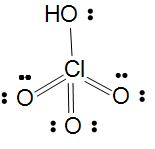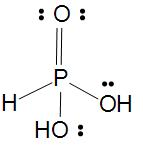QuestionAnswers

# Draw the structures of the following:(1) $HCl{{O}_{4}}$ (2) ${{H}_{3}}P{{O}_{3}}$Hint: Perchloric acid is monobasic so there will be one $Cl-OH$ bond and Phosphorous acid is dibasic so there will be two $P-OH$ bonds. It has tetrahedral geometry just like methane with bond angle 109.8.

Perchloric acidBasicity of oxoacids of Cl is equal to the number of $Cl-OH$ bonds in the molecule.
In Perchloric acid its basicity is 1 as there is one $Cl-OH$ bond in the molecule and three $Cl-O$ bonds due to which it is oxidizing power is less. Here Perchloric acid is monobasic and the halogen(chlorine) is $s{{p}_{3}}$ hybridized.
Halogens forms several oxyacids such as $HOX$, $HOXO$, $HOX{{O}_{2}}$, $HOX{{O}_{3}}$.
Order of acidic strength of oxoacids of the same halogen is
$HCl{{O}_{4}}$>$HCl{{O}_{3}}$>$HCl{{O}_{2}}$>$HCl{{O}_{{}}}$ it’s like this because acidic strength of oxoacids of same halogen increases when there oxidation state increases.
This following trend can also be seen from the stability of anion produced more stable is the conjugate base more is the acidic character.
Oxidizing power of the oxoacids of chlorine is
$HCl{{O}_{4}}$<$HCl{{O}_{3}}$<$HCl{{O}_{2}}$<$HCl{{O}_{{}}}$.

Phosphorous acidBasicity of oxoacids of P is equal to the number of $P-OH$ bonds in the molecule.
In Phosphorous acid its basicity is 2 as there is two $P-OH$ bond in the molecule which we can also say as dibasic and one $P=O$ bond and one $P-H$ bond which help to be as a strong reducing agents but Phosphorous acid is a weak reducing agent as we can see there is only one $P-H$ bond.
Oxidation state of phosphorus is tetrahedrally surrounded by other atoms.
Phosphorous acid is Dibasic due to the presence of two replaceable hydrogen.
On heating, ${{H}_{3}}P{{O}_{3}}$ undergoes disproportionation reaction to form ${{H}_{3}}P{{O}_{3}}$ and $P{{H}_{3}}$
$4{{H}_{3}}P{{O}_{3}}\xrightarrow{\Delta }3{{H}_{3}}P{{O}_{4}}+P{{H}_{3}}$

Note:
- Phosphorous acid is triprotic as we can judge by the given formula ${{H}_{3}}P{{O}_{3}}$.
- Perchloric acid solids made up of perchlorate anion.

Structure of Acetylene - The Triple BondsThe Idea of TimeWhat are the Functions of the Human Skeletal System?The Ghat of the Only World SummaryThe Perimeter of Rectangle FormulaDark Side of the MoonMeasuring the Rate of Change of MotionStructure of AtomThe Converse of Pythagoras TheoremBiomes of the WorldImportant Questions for CBSE Class 11 Chemistry Chapter 2 - Structure of AtomImportant Questions for CBSE Class 11 English Snapshots Chapter 1 - The Summer of the Beautiful White HorseImportant Questions for CBSE Class 11 Chemistry Chapter 1 - Some Basic Concepts of ChemistryImportant Questions for CBSE Class 11 Chemistry Chapter 11 - The p-Block ElementsImportant Questions for CBSE Class 11 Chemistry Chapter 10 - The s-Block ElementsImportant Questions for CBSE Class 12 Chemistry Chapter 1 - The Solid StateNCERT Books Free Download for Class 11 Chemistry Chapter 2 - Structure of AtomImportant Questions for CBSE Class 11 English Snapshots Chapter 2 - The AddressImportant Questions for CBSE Class 11 Biology Chapter 1 - The Living WorldImportant Questions for CBSE Class 9 Science Chapter 4 - Structure of The AtomChemistry Question Paper for CBSE Class 12 - 2016 Set 1 EChemistry Question Paper for CBSE Class 12 - 2016 Set 1 SChemistry Question Paper for CBSE Class 12 - 2016 Set 1 NChemistry Question Paper for CBSE Class 12 - 2016 Set 1 CChemistry Question Paper for CBSE Class 12CBSE Class 12 Chemistry Question Paper 2020Chemistry Question Paper for CBSE Class 12 - 2013Chemistry Question Paper for CBSE Class 12 - 2015CBSE Class 12 Chemistry Question Paper 2019 - Free PDFCBSE Class 12 Chemistry Question Paper 2017 - Free PDFNCERT Solutions for Class 11 chemistry chapter 2 – Structure of AtomLakhmir Singh Chemistry Class 9 Solutions Chapter 4 - Structure Of The AtomNCERT Solutions for Class 11 Chemistry Chapter 2 Structure of Atom In HindiRD Sharma Class 11 Solutions Chapter 1 - Sets (Ex 1.2) Exercise 1.2RD Sharma Class 11 Solutions Chapter 2 - Relations (Ex 2.1) Exercise 2.1NCERT Solutions for Class 11 Maths Chapter 2 Relations and Functions (Ex 2.1) Exercise 2.1NCERT Solutions for Class 11 Maths Chapter 1 Sets (Ex 1.2) Exercise 1.2NCERT Solutions for Class 11 Chemistry Chapter 1 Some Basic Concepts of Chemistry in HindiNCERT Solutions Class 11 English Woven Words Prose Chapter 4 The Adventure of the Three GarridebsNCERT Solutions Class 11 Chemistry Chapter 1